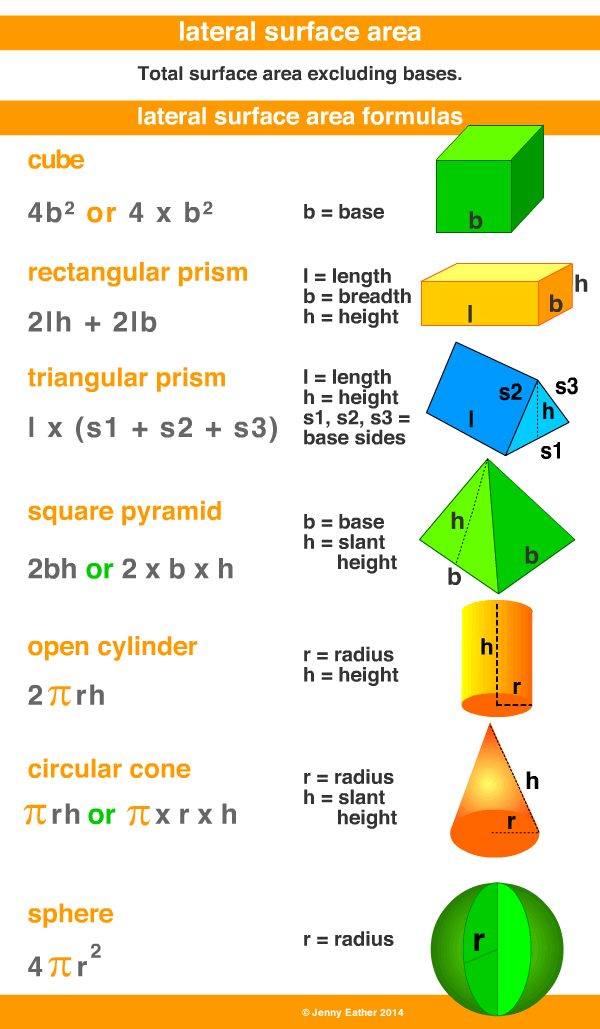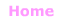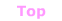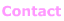Aa Bb Cc Dd Ee Ff Gg Hh Ii Jj Kk Ll Mm Nn Oo Pp Qq Rr Ss Tt Uu Vv Ww Xx Yy Zz

Ll

lateral surface area

• total area of the surface of a three-dimensional object,
NOT including the bases.

EXAMPLES:Lateral Surface Area Formulas

Lateral surface area of a cube = 4b2 ~ b is base
Lateral surface area of a sphere is 4πr2 ~ π is pi, r is radius
Lateral surface area of a cone = π × r × l ~ π is pi, r is radius, l is slant height
Lateral surface area of a cylinder = 2πrh ~ π is pi, r is radius, h is height
Lateral surface area of right pyramids = n × area of lateral faces
~ n is number of sides on the base
Lateral surface area of prisms = n × area of each rectangle
~ n is number of sides on the base© Jenny Eather 2014. All rights reserved.